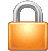• Check is null or not

hi freinds,

i am using following code to get last number from table

`declare @LastValue as varchar(10) declare @sr as varchar(10)declare @Prefix as varchar(50) declare @Temp as int set @sr='GG55000004'set @prefix = substring(@sr,0,5)select top 1 case when substring(sr,5,7) =null then 'TEST1' else 'TEST2' end from table1 where substring(sr,0,5)=substring(@sr,0,5) order by cast(substring(sr,5,7) as bigint) desc`

i am getting 'TEST2' when there are some records in table,
but if there are no record as it is running first time,
its return nothing as i expected 'TEST1'
please tell me where i am wrong.

thank you
• Hi There--

SQL null checking:
ColumNamr IS NULL

select top 1 case when substring(sr,5,7) is null then 'TEST1' else 'TEST2' end from table1 where substring(sr,0,5)=substring(@sr,0,5) order by cast(substring(sr,5,7) as bigint) desc

Thnx,
Ram

• Hi,

IF you want check NULL then use IS NULL and if field is empty use some single quote rewrite that last line like below query

`select top 1 case when substring(sr,5,7) is null then 'TEST1' case when substring(sr,5,7) = '' then 'TEST1' else 'TEST2' end from table1 where substring(sr,0,5)=substring(@sr,0,5) order by cast(substring(sr,5,7) as bigint) desc`

Regards
N.Ravindran

• hi try this code

`declare @LastValue as varchar(10) declare @sr as varchar(10)declare @Prefix as varchar(50) declare @Temp as int set @sr='GG55000004'set @prefix = substring(@sr,0,5)select top 1 case when substring(sr,5,7) = '' then 'TEST1' else 'TEST2' end from table1 where substring(sr,0,5)=substring(@sr,0,5) order by cast(substring(sr,5,7) as bigint) desc`

• Hi.

If you want to check Null value then we can not use Eqaul(=) sign in sql server.
Insterad of Equal we use is keyword.
syntax =>
` select * from tableName where ColumnName condition`

Example =>
` select * from table1 where is null`

`declare @LastValue as varchar(10) declare @sr as varchar(10)declare @Prefix as varchar(50) declare @Temp as int set @sr='GG55000004'set @prefix = substring(@sr,0,5)select top 1 case when substring(sr,5,7) is null then 'TEST1' else 'TEST2' end from table1 where substring(sr,0,5)=substring(@sr,0,5) order by cast(substring(sr,5,7) as bigint) desc`
•This thread is locked for new responses. Please post your comments and questions as a separate thread.